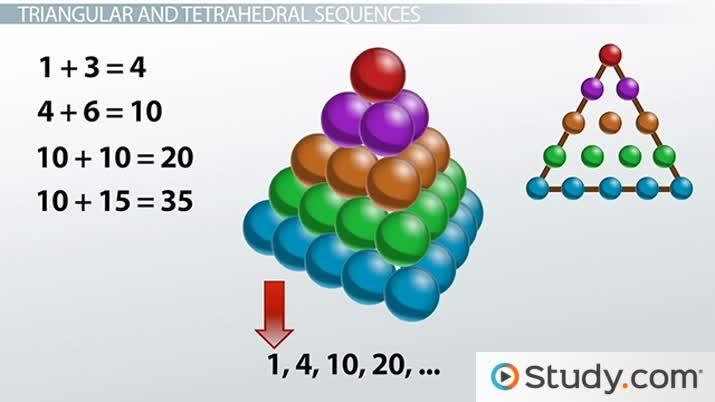Special Sequences and How They Are Generated

Lesson Transcript
Instructor
Yuanxin (Amy) Yang Alcocer

Amy has a master's degree in secondary education and has been teaching math for over 9 years. Amy has worked with students at all levels from those with special needs to those that are gifted.

Expert Contributor
Elaine Chan

Dr. Chan has taught computer and college level physics, chemistry, and math for over eight years. Dr. Chan has a Ph.D. in Chemistry from U. C. Berkeley, an M.S. Physics plus 19 graduate Applied Math credits from UW, and an A.B. with honors from U.C .Berkeley in Physics.

Special sequences are a string of numbers that have a unique pattern to them. Discover how special sequences are generated and some types such as triangular, tetrahedral, cube, square, and fibonacci sequences. Updated: 10/06/2021

Special Sequences

Sequences are a string of numbers. When a sequence has a unique pattern to it, we call it a special sequence. You can even think of our number line, our counting numbers, as a special sequence. You learned it when you were little, so you can count - one, two, three, and so on. What is the pattern to our counting numbers? Our counting numbers have the pattern that each number is equal to the previous number plus one.

Besides our counting numbers, there are actually quite a few special sequences out there. We will talk about the more popular ones in this video lesson. It's good to know about these special sequences because you will actually see and use these numbers often in math problems.An error occurred trying to load this video.

Try refreshing the page, or contact customer support.

Coming up next: Introduction to Groups and Sets in Algebra

You're on a roll. Keep up the good work!

Replay
Your next lesson will play in 10 seconds
• 0:02 Special Sequences
• 0:43 Triangular and…
• 2:19 Square and Cube Sequences
• 3:06 Fibonacci Sequence
• 4:08 Lesson Summary
Save Save

Want to watch this again later?

Timeline
Autoplay
Autoplay
Speed Speed

Triangular and Tetrahedral Sequences

We begin with triangular and tetrahedral sequences. A triangular sequence is a sequence that gives you the numbers needed to form a triangle. Think of yourself building triangles with marbles. You begin with one marble. To get the next triangle, you would need to add two more marbles. So now, you have a total of three marbles.

To grow your triangle again, how many marbles would you need? You would need three more marbles to form the new base of your triangle. Now you have six marbles. Your sequence is now 1, 3, 6... Do you see the pattern? To make our triangles, we add two to our first term, three to our second term, and so on. We continue our sequence by adding four to the third term to get 10, then adding five to the fourth term to 15. We now have 1, 3, 6, 10, and 15.

Our next special sequence is a tetrahedral sequence. This is a sequence of the number of units needed to form a tetrahedron, a triangular pyramid. Think of the triangles we just built as each layer of our tetrahedron. So, a tetrahedron of height one will have one marble. For a height of two, it will have one plus three marbles, or four marbles. For a height of three, it will have four plus six, or ten, marbles. We are essentially adding our triangular numbers up with each term. So, our series looks like 1, 4, 10, 20, etc.

Square and Cube Sequences

Our next group of special sequences includes the square and cube sequences. The square sequence is a sequence in which each term is the square of the corresponding number on the number line. So, the first term is one squared. The second term is two squared; the third term is three squared. So, this sequence looks like 1, 4, 9, 16, etc.

The cube sequence is a sequence in which each term is the cube of the corresponding number on the number line. So, the first term here is one cubed; the second term, two cubed; the third term, three cubed; and so on. This sequence looks like 1, 8, 27, 64, etc.

To unlock this lesson you must be a Study.com Member.

Practice Sequences

Problem 1:

Find a formula for the general term of the sequence 1/2, 1/4, 1/8, 1/16.., assuming the pattern of the first few terms continues.

Problem 2:

Find a formula for the general term of the sequence 3/16, 4/25, 5/36, 6/49..., assuming the pattern of the first few terms continues.

Problem 3:

What is the formula for the nth term in an arithmetic sequence: a1 , a1 + d, a1 + 2d, a1 + 3d, ...?

Problem 4:

Consider the geometric sequence 3, 6,12, 24, 48, ... Find a formula for an.

Problem 5:

A natural number (1,2,3,4,... ) is called a prime number if it is greater than 1 and cannot be written as the product of two smaller natural numbers.

What are the missing numbers in the following sequence of prime numbers?

2, 3, 5, 7, 11, _____, 17, 19, 23, 29, 31, _____, 41, 43, 47, 53, 59, 61, _____, 71, 73, 79, _____, 89, 97,...

1/2n

(n+2)/(n+3)2

In an arithmetic sequence with steps of size d, an = a1+(n + 1) d

The ratio between successive terms is r. The nth term in the sequence is:

an = rn-1 a1 = 2n-1 ( 3 ).

13, 37, 67, 83

Register to view this lesson

Are you a student or a teacher?